# CS 179 GPU Programming Lecture 8 Last time

• Slides: 38
Download presentationCS 179: GPU Programming Lecture 8Last time • GPU-accelerated: – – Reduction Prefix sum Stream compaction Sorting (quicksort)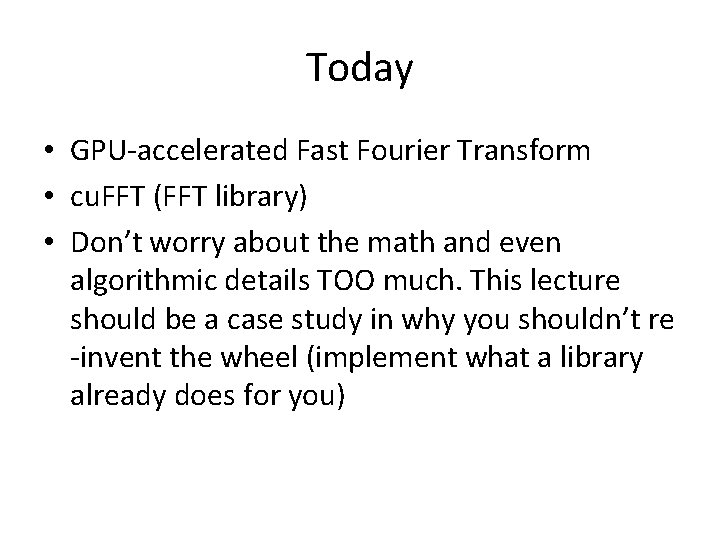Today • GPU-accelerated Fast Fourier Transform • cu. FFT (FFT library) • Don’t worry about the math and even algorithmic details TOO much. This lecture should be a case study in why you shouldn’t re -invent the wheel (implement what a library already does for you)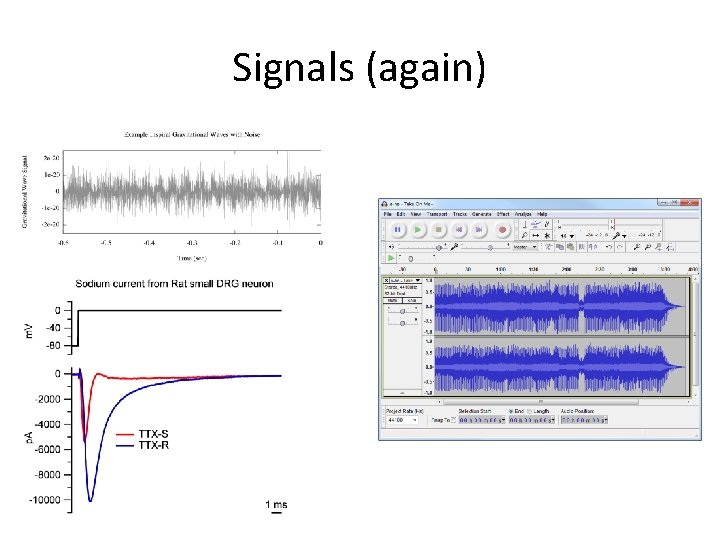Signals (again)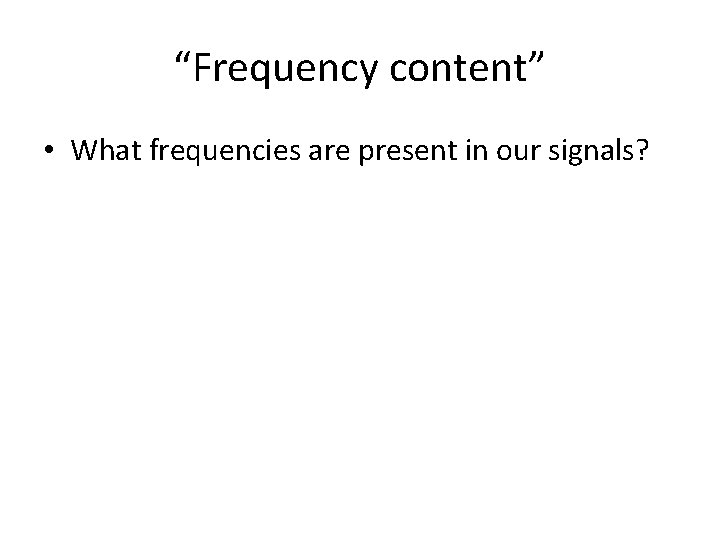“Frequency content” • What frequencies are present in our signals?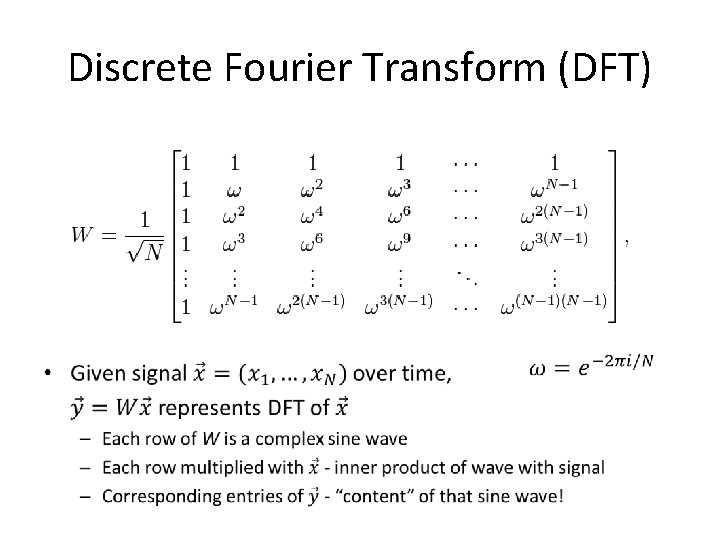Discrete Fourier Transform (DFT) •Solid line = real part Dashed line = imaginary part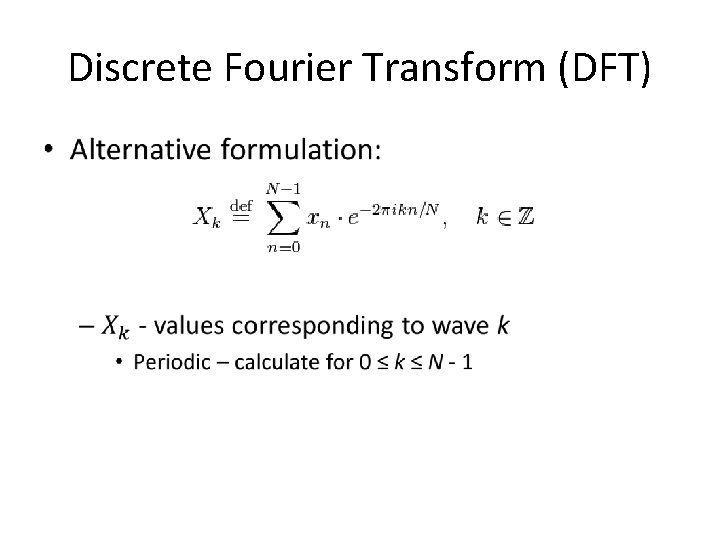Discrete Fourier Transform (DFT) •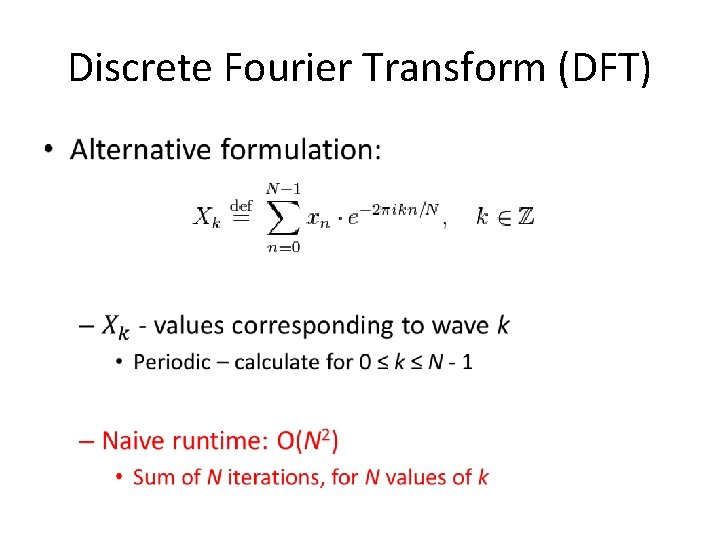Discrete Fourier Transform (DFT) •Discrete Fourier Transform (DFT) •Roots of unityDiscrete Fourier Transform (DFT) • Number of distinct values: N, not N 2!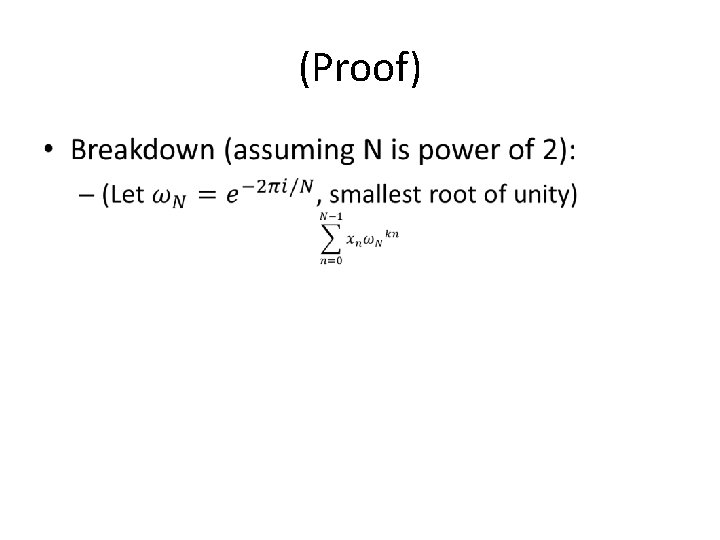(Proof) •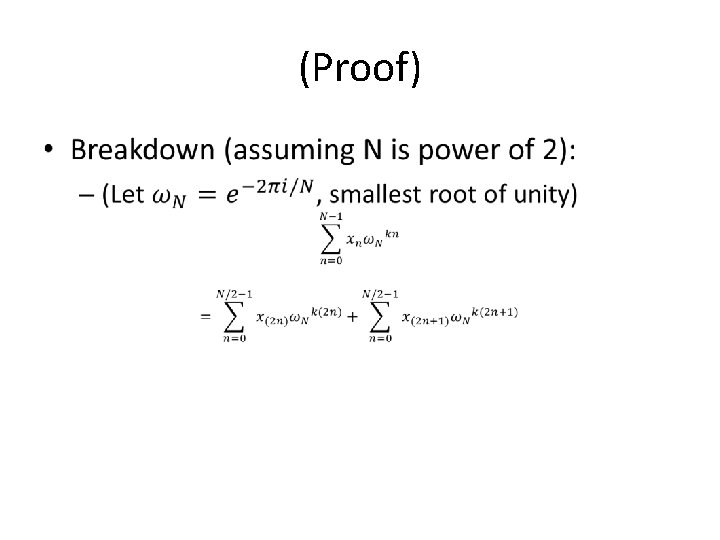(Proof) •(Proof) •(Proof) •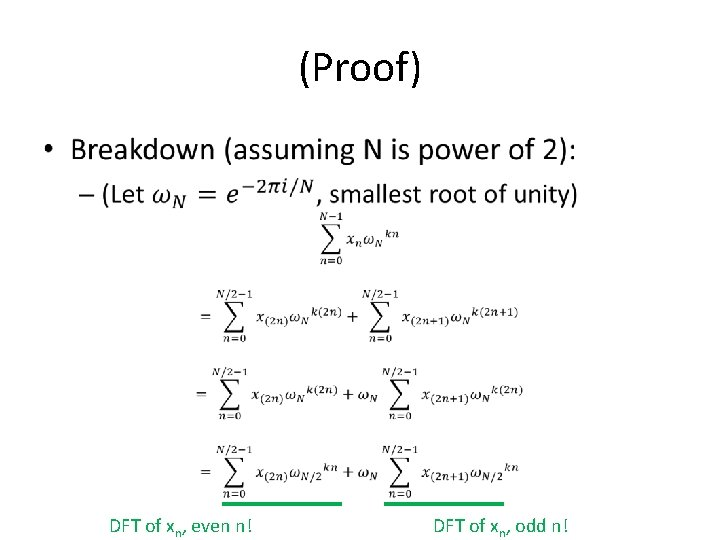(Proof) • DFT of xn, even n! DFT of xn, odd n!(Divide-and-conquer algorithm) Recursive-FFT(Vector x): if x is length 1: return x x_even <- (x 0, x 2, . . . , x_(n-2) ) x_odd <- (x 1, x 3, . . . , x_(n-1) ) y_even <- Recursive-FFT(x_even) y_odd <- Recursive-FFT(x_odd) for k = 0, …, (n/2)-1: y[k] <- y_even[k] + wk * y_odd[k] y[k + n/2] <- y_even[k] - wk * y_odd[k] return y(Divide-and-conquer algorithm) Recursive-FFT(Vector x): if x is length 1: return x x_even <- (x 0, x 2, . . . , x_(n-2) ) x_odd <- (x 1, x 3, . . . , x_(n-1) ) y_even <- Recursive-FFT(x_even) y_odd <- Recursive-FFT(x_odd) for k = 0, …, (n/2)-1: y[k] <- y_even[k] + wk * y_odd[k] y[k + n/2] <- y_even[k] - wk * y_odd[k] return y T(n/2) O(n)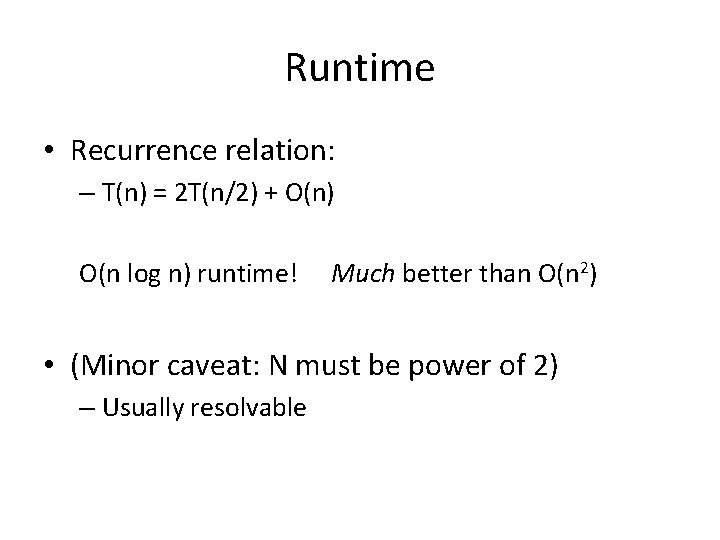Runtime • Recurrence relation: – T(n) = 2 T(n/2) + O(n) O(n log n) runtime! Much better than O(n 2) • (Minor caveat: N must be power of 2) – Usually resolvable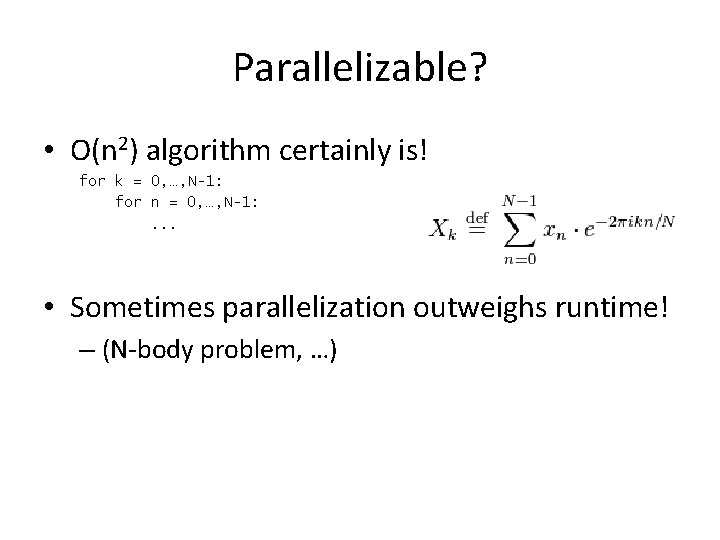Parallelizable? • O(n 2) algorithm certainly is! for k = 0, …, N-1: for n = 0, …, N-1: . . . • Sometimes parallelization outweighs runtime! – (N-body problem, …)Recursive index tree x 0, x 1, x 2, x 3, x 4, x 5, x 6, x 7 x 0, x 2, x 4, x 6 x 0, x 4 x 0 x 1, x 3, x 5, x 7 x 2, x 6 x 4 x 2 x 1, x 5 x 6 x 1 x 3, x 7 x 5 x 3 x 7Recursive index tree x 0, x 1, x 2, x 3, x 4, x 5, x 6, x 7 x 0, x 2, x 4, x 6 x 0, x 4 x 0 x 1, x 3, x 5, x 7 x 2, x 6 x 4 x 2 x 1, x 5 x 6 x 1 x 3, x 7 x 5 x 3 Order? x 7Bit-reversal order 0 4 2 6 1 5 3 7 000 100 010 110 001 101 011 111Bit-reversal order 0 4 2 6 1 5 3 7 000 reverse of… 100 010 110 001 101 011 111 000 001 010 011 100 101 110 111 0 1 2 3 4 5 6 7(Divide-and-conquer algorithm review) Recursive-FFT(Vector x): if x is length 1: return x x_even <- (x 0, x 2, . . . , x_(n-2) ) x_odd <- (x 1, x 3, . . . , x_(n-1) ) y_even <- Recursive-FFT(x_even) y_odd <- Recursive-FFT(x_odd) for k = 0, …, (n/2)-1: y[k] <- y_even[k] + wk * y_odd[k] y[k + n/2] <- y_even[k] - wk * y_odd[k] return y T(n/2) O(n)Iterative approach x 0, x 1, x 2, x 3, x 4, x 5, x 6, x 7 Stage 3 x 0, x 2, x 4, x 6 x 1, x 3, x 5, x 7 Stage 2 x 0, x 4 x 2, x 6 x 1, x 5 x 3, x 7 Stage 1 x 0 x 4 x 2 x 6 x 1 x 5 x 3 Bit-reversed accesses (a la sum reduction) x 7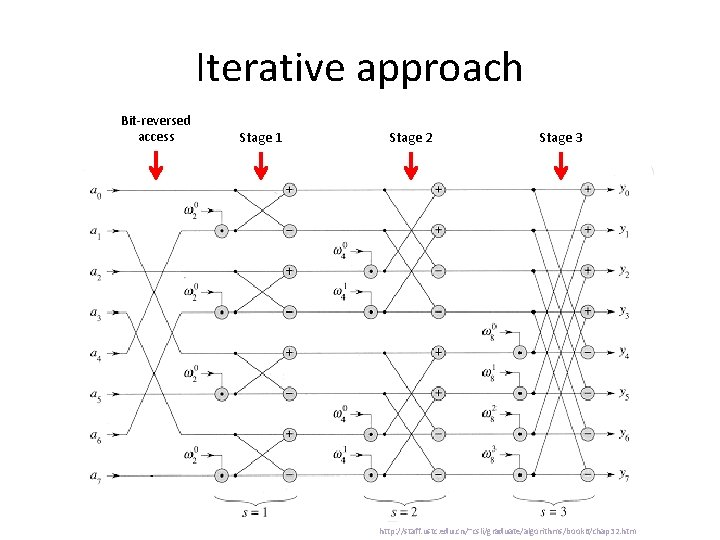Iterative approach Bit-reversed access Stage 1 Stage 2 Stage 3 http: //staff. ustc. edu. cn/~csli/graduate/algorithms/book 6/chap 32. htm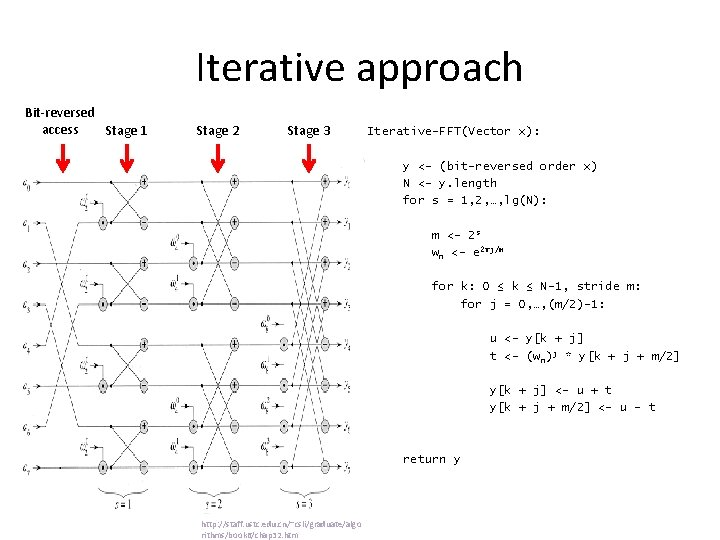Iterative approach Bit-reversed access Stage 1 Stage 2 Stage 3 Iterative-FFT(Vector x): y <- (bit-reversed order x) N <- y. length for s = 1, 2, …, lg(N): m <- 2 s wn <- e 2πj/m for k: 0 ≤ k ≤ N-1, stride m: for j = 0, …, (m/2)-1: u <- y[k + j] t <- (w n)j * y[k + j + m/2] y[k + j] <- u + t y[k + j + m/2] <- u - t return y http: //staff. ustc. edu. cn/~csli/graduate/algo rithms/book 6/chap 32. htmCUDA approach Bit-reversed access Stage 1 Stage 2 Stage 3 http: //staff. ustc. edu. cn/~csli/graduate/algorithms/book 6/chap 32. htm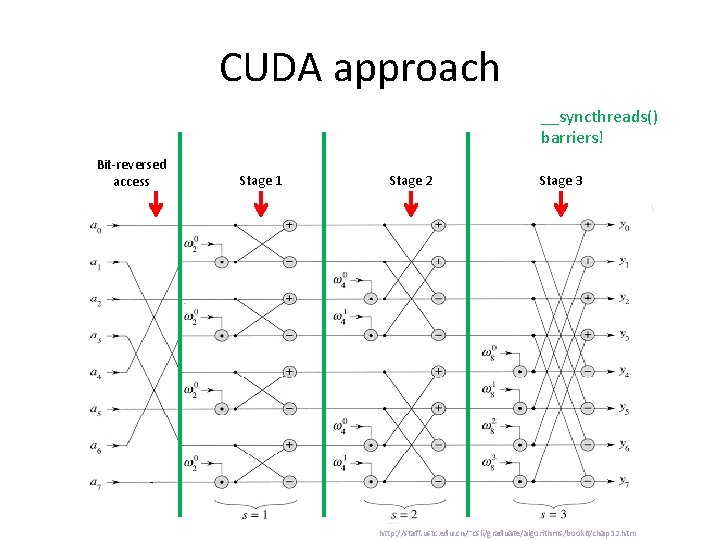CUDA approach __syncthreads() barriers! Bit-reversed access Stage 1 Stage 2 Stage 3 http: //staff. ustc. edu. cn/~csli/graduate/algorithms/book 6/chap 32. htmCUDA approach Non-coalesced memory access!! Bit-reversed access __syncthreads() barriers! Stage 1 Stage 2 Stage 3 http: //staff. ustc. edu. cn/~csli/graduate/algorithms/book 6/chap 32. htm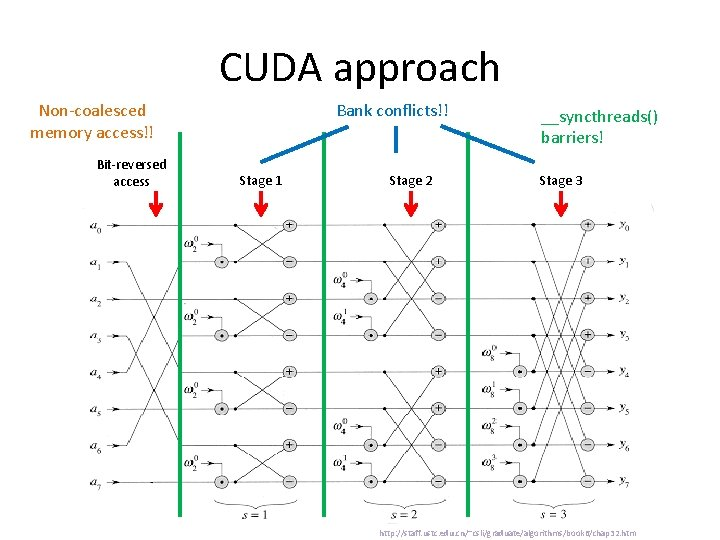CUDA approach Non-coalesced memory access!! Bit-reversed access Bank conflicts!! Stage 1 Stage 2 __syncthreads() barriers! Stage 3 http: //staff. ustc. edu. cn/~csli/graduate/algorithms/book 6/chap 32. htm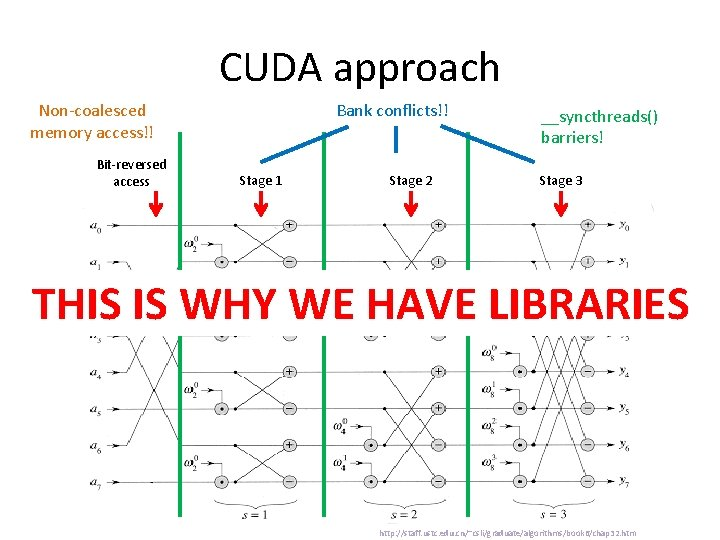CUDA approach Non-coalesced memory access!! Bit-reversed access Bank conflicts!! Stage 1 Stage 2 __syncthreads() barriers! Stage 3 THIS IS WHY WE HAVE LIBRARIES http: //staff. ustc. edu. cn/~csli/graduate/algorithms/book 6/chap 32. htm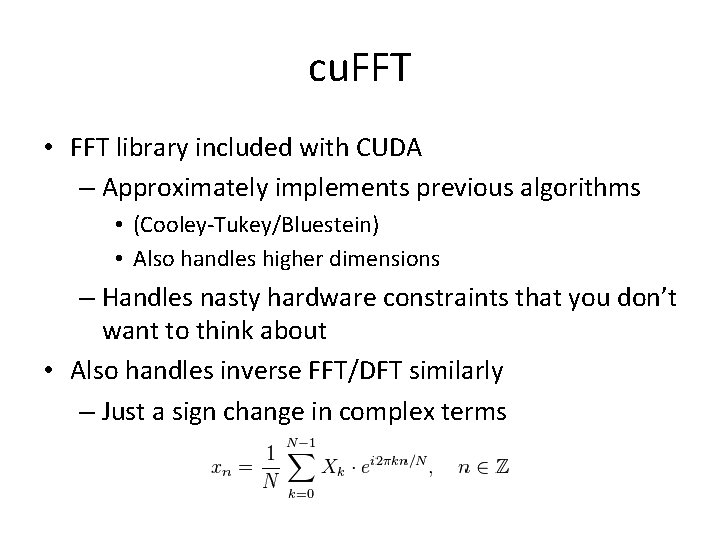cu. FFT • FFT library included with CUDA – Approximately implements previous algorithms • (Cooley-Tukey/Bluestein) • Also handles higher dimensions – Handles nasty hardware constraints that you don’t want to think about • Also handles inverse FFT/DFT similarly – Just a sign change in complex termscu. FFT 1 D example Correction: Remember to use cufft. Destroy(plan) when finished with transforms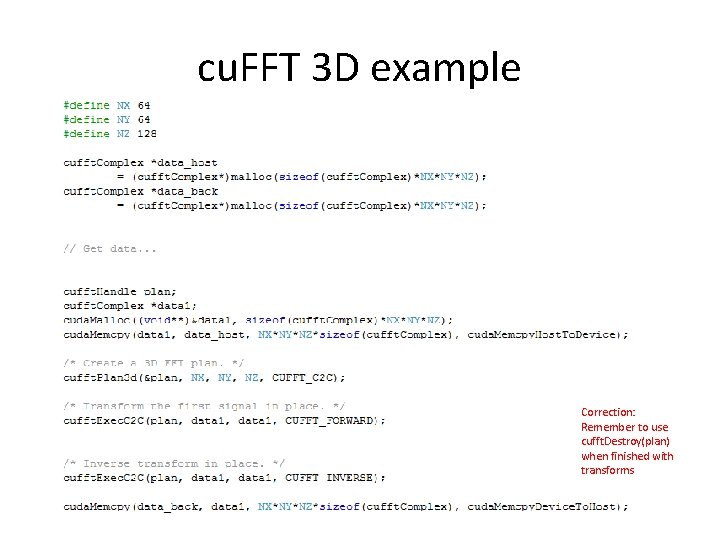cu. FFT 3 D example Correction: Remember to use cufft. Destroy(plan) when finished with transforms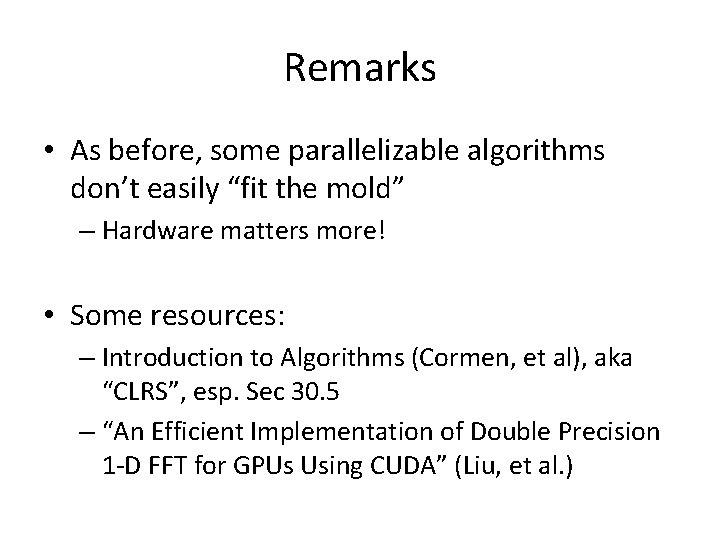Remarks • As before, some parallelizable algorithms don’t easily “fit the mold” – Hardware matters more! • Some resources: – Introduction to Algorithms (Cormen, et al), aka “CLRS”, esp. Sec 30. 5 – “An Efficient Implementation of Double Precision 1 -D FFT for GPUs Using CUDA” (Liu, et al. )